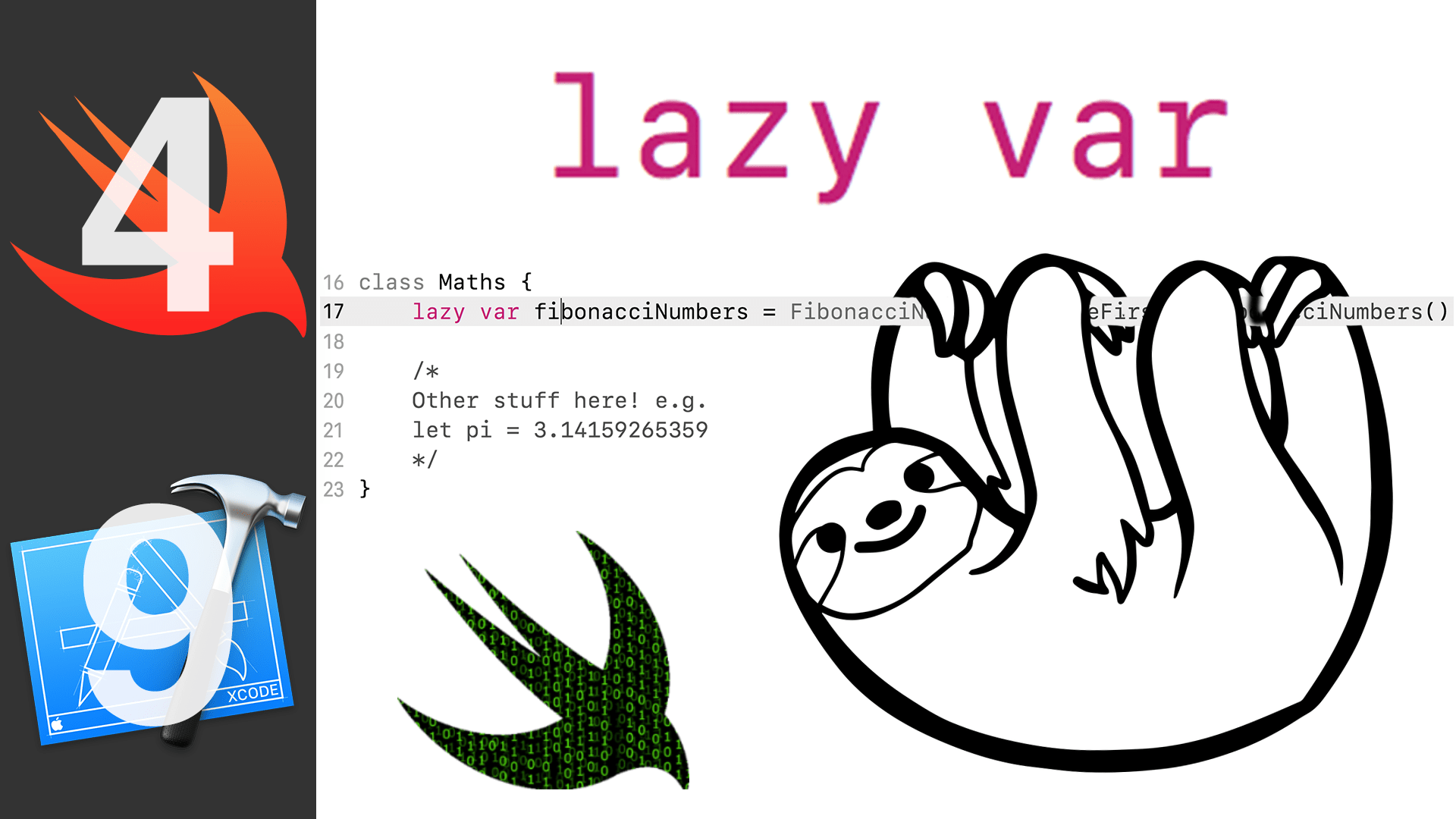# Lazy Stored Property – DevTip

Chances are that you have used or at least have seen the lazykeyword used before. However, you have never stopped to think about what are the true capabilities of a lazy stored property. I want to tell you about what lazy properties are, why they exist and how you can take advantage of them.

# Ground Work

The initial value of lazy stored properties isn’t calculated and assigned until you call the property. You can think of this as an on-demand property, it doesn’t exist in the memory until you call it.

A lazy property must always be a variable since constants must always have an initial value at the instance initialization.

Lazy stored properties aren’t thread safe!
If a property marked with the lazy modifier is accessed by multiple threads simultaneously and the property has not yet been initialized, there is no guarantee that the property will be initialized only once.

# When do you use a lazy stored property?

1. When the property is dependent on an outside source and the value may not be known until after the initialization is complete (asynchronous calls like web request)
2. If the initial value of the property requires a computationally expensive setup that should not be performed until the value is needed
3. To avoid optionals AKA non-optionaloptionss
4. Keep initializers clean by deferring some setup until after later in the life-cycle of the object

# Using lazy stored properties

The simplest way to implement a lazy stored property is by putting the lazy keyword before a variable with a default value. This means that the property won’t be initialized during the type’s initialization process. The initial value is only going to be assigned when the property is called.

class SomeClass {
lazy var storedProp = ""
}

let instance = SomeClass()
instance.storedProp // The property isn't initialized until this point


Another way to initialize a lazy property is by using a method. This is useful if you want to separate the property and the initialization or if you have a lengthy and complex initialization. For this, you need to create an initialization method and call that on the property.
In the example, I used the Fibonacci numbers as they can be computationally heavy. I made this example vary unoptimized to make a point, this isn’t how you would implement this in real life.

class SomeClass {
lazy var fibonacciNumbers = self.createFirstFewFibonacciNumbers()

let currentNum = fibonacciNumbers.last!
let prevNum = fibonacciNumbers[fibonacciNumbers.count - 2]

let newNum = currentNum + prevNum
fibonacciNumbers.append(newNum)
}

private func createFirstFewFibonacciNumbers() -> [Int] {
var fibonacciNumbers = [0, 1, 1]
for index in 2...8 {
let currentNum = fibonacciNumbers[index]
let prevNum = fibonacciNumbers[index - 1]

let newNum = currentNum + prevNum
fibonacciNumbers.append(newNum)
}
return fibonacciNumbers
}
}

let instance = SomeClass()


If you’re like me and you like compactness when it doesn’t compromise readability then you can move all the code that is defined in the initialization method into an auto-executing closure.

class SomeClass {
lazy var fibonacciNumbers: [Int] = {
var fibonacciNumbers = [0, 1, 1]
for index in 2...8 {
let currentNum = fibonacciNumbers[index]
let prevNum = fibonacciNumbers[index - 1]

let newNum = currentNum + prevNum
fibonacciNumbers.append(newNum)
}
return fibonacciNumbers
}()

let currentNum = fibonacciNumbers.last!
let prevNum = fibonacciNumbers[fibonacciNumbers.count - 2]

let newNum = currentNum + prevNum
fibonacciNumbers.append(newNum)
}
}

let instance = SomeClass()


There is one more way you can assign an initial value to a lazy property, that is using type methods. This method capitalizes on the single responsibility principle and type decoupling. This is the most tedious of all and I only recommend it if you actually find a good use for it.
For instance, in my example, I have 2 classes. One which is all about the Fibonacci numbers and the other one is a general maths class.

class FibonacciNumbers {
static func createFirstFewFibonacciNumbers() -> [Int] {
var fibonacciNumbers = [0, 1, 1]
for index in 2...8 {
let currentNum = fibonacciNumbers[index]
let prevNum = fibonacciNumbers[index - 1]

let newNum = currentNum + prevNum
fibonacciNumbers.append(newNum)
}
return fibonacciNumbers
}
}

class Maths {
lazy var fibonacciNumbers = FibonacciNumbers.createFirstFewFibonacciNumbers()

/*
Other stuff here! e.g.
let pi = 3.14159265359
*/
}

let instance = SomeClass()
instance.fibonacciNumbers


# Conclusion

Lazy stored properties can be very useful for optimizing RAM and CPU usage and keeping our code base readable.

There is no sign of performance difference between any of the above methods of initializing the property.